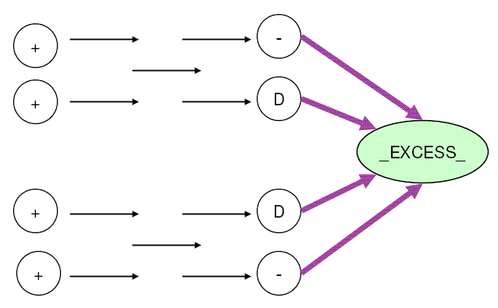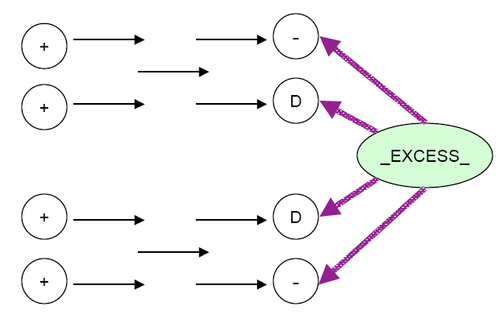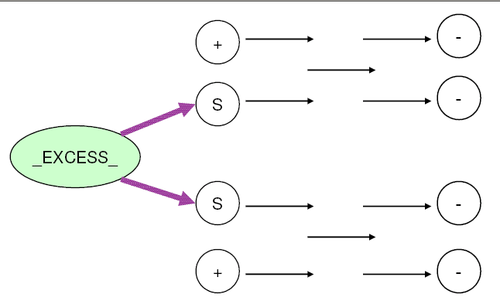### The EXCESS=DEMAND Option

If you specify the EXCESS=DEMAND option, then there are three possible scenarios to deal with:

Case 1: No Node with Missing S Supply, THRUNET Not Specified (see Figure 6.36)

Supply the excess demand to all demand nodes directly.

Figure 6.36: Nodes with Missing D DemandCase 2: Some Nodes with Missing S Supply, THRUNET Not Specified (see Figure 6.37)

Supply the excess demand by the nodes that have a missing S supply value. If a node has a missing S supply value, then the amount it supplies is determined by optimization. For a supply node with a positive `supdem` value, that value is equal to the sum of flows on all actual arcs directed away from that node.

Figure 6.37: Nodes with Missing S SupplyCase 3: THRUNET Specified (see Figure 6.38)

Supply the excess demand by all supply nodes. If a node has a positive `supdem` value, that value is the lower bound on the sum of flows on all actual arcs directed away from that node. If a node has a missing S supply value, then the amount it supplies is determined by optimization.

Figure 6.38: Nodes with Missing S Supply, THRUNET Specified